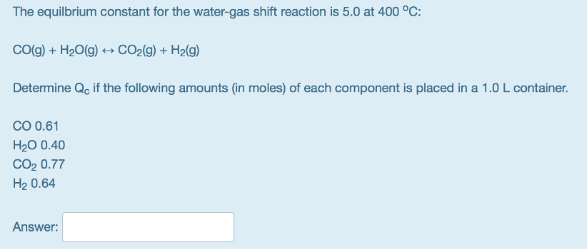# Problem: The equilbrium constant for the water-gas shift reaction is 5.0 at 400 °C: CO(g) + H2O(g) &lt;—&gt; CO2(g) + H2(g) Determine Qc, if the following amounts (in moles) of each component is placed in a 1.0 L container. CO 0.61 H2O 0.40 CO2 0.77 H2 0.64

###### FREE Expert Solution
97% (75 ratings)###### Problem Details

The equilbrium constant for the water-gas shift reaction is 5.0 at 400 °C:

CO(g) + H2O(g) <—> CO2(g) + H2(g)

Determine Qc, if the following amounts (in moles) of each component is placed in a 1.0 L container.

CO 0.61

H2O 0.40

CO2 0.77

H2 0.64